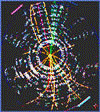## Research Papers in Physics and Astronomy## Kenneth Bloom Publications

#### Date of this Version

2007

Published in J. Phys. G: Nucl. Part. Phys. 34 (2007) 2457–2544. © 2007 IOP Publishing Ltd

#### Abstract

We report the first measurements of inclusive W and Z boson cross-sections
times the corresponding leptonic branching ratios for pp collisions at √s = 1.96 TeV based on the decays of the W and Z bosons into electrons and muons. The data were recorded with the CDF II detector at the Fermilab Tevatron and correspond to an integrated luminosity of 72.0 ± 4.3 pb−1. We test e-μ lepton universality in W decays by measuring the ratio of the W → μν to W → eν cross sections and determine a value of 0.991 ±0.004(stat.) ± 0.011(syst.) for the ratio of W − L − ν couplings (gμ/ge). Since there is no sign of non-universality, we combine our cross-section measurements in the different lepton decay modes and obtain σW × Br(pp → W → Lν) = 2.749 ±
0.010(stat.) ± 0.053(syst.) ± 0.165(lum.) nb and σγ/Z× Br(pp → γ/Z →LL) = 254.9 ± 3.3(stat.) ± 4.6(syst.) ± 15.2(lum.) pb for dilepton pairs in the mass range between 66 GeV/c2 and 116 GeV/c2. We compute the ratio R of the W → Lν to Z → LL cross sections taking all correlations among channels into account and obtain R = 10.84 ± 0.15(stat.) ± 0.14(syst.) including a correction for the virtual photon exchange component in our measured γ/Z → LL cross section. Based on the measured value of R, we extract values for the W leptonic branching ratio, Br(W → Lν) = 0.1082 ± 0.0022; the total width of the W boson, Γ(W) = 2092 ± 42 MeV; and the ratio of W and Z boson total widths, Γ(W)/ Γ(Z) = 0.838 ± 0.017. In addition, we use our extracted value of Γ(W) whose value depends on various electroweak parameters and certain CKM matrix elements to constrain the Vcs CKM matrix element, |Vcs| = 0.976 ± 0.030.

COinS